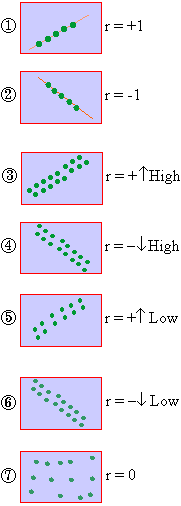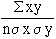Support the Monkey! Tell All your Friends and TeachersHome MonkeyNotes Printable Notes Digital Library Study Guides Study Smart Parents Tips College Planning Test Prep Fun Zone Help / FAQ How to Cite Request a New Title

6.5 Methods Of Determining Correlation

We shall consider the following most commonly used methods.(1) Scatter Plot (2) Kar Pearsons coefficient of correlation (3) Spearmans Rank-correlation coefficient.

1) Scatter Plot ( Scatter diagram or dot diagram ): In this method the values of the two variables are plotted on a graph paper. One is taken along the horizontal ( (x-axis) and the other along the vertical (y-axis). By plotting the data, we get points (dots) on the graph which are generally scattered and hence the name Scatter Plot.

The manner in which these points are scattered, suggest the degree and the direction of correlation. The degree of correlation is denoted by  r  and its direction is given by the signs positive and negative.i) If all points lie on a rising straight line the correlation is perfectly positive and r = +1 (see fig.1 )

ii) If all points lie on a falling straight line the correlation is perfectly negative and r = -1 (see fig.2)

iii) If the points lie in narrow strip, rising upwards, the correlation is high degree of positive (see fig.3)

iv) If the points lie in a narrow strip, falling downwards, the correlation is high degree of negative (see fig.4)

v) If the points are spread widely over a broad strip, rising upwards, the correlation is low degree positive (see fig.5)

vi) If the points are spread widely over a broad strip, falling downward, the correlation is low degree negative (see fig.6)

vii) If the points are spread (scattered) without any specific pattern, the correlation is absent. i.e. r = 0. (see fig.7)

Though this method is simple and is a rough idea about the existence and the degree of correlation, it is not reliable. As it is not a mathematical method, it cannot measure the degree of correlation.Your browser does not support the IFRAME tag.

2) Karl Pearsons coefficient of correlation: It gives the numerical expression for the measure of correlation. it is noted by  r . The value of  r  gives the magnitude of correlation and sign denotes its direction. It is defined as

r =whereN = Number of pairs of observation

Index

6. 1 Introduction
6. 2 Correlation
6. 3 Types of Correlation
6. 4 Degrees of Correlation
6. 5 Methods of determining correlation
6. 6 Coefficients of Correlation for Bivariate Grouped Data
6. 7 Probable Error
6. 8 Rank Correlation Coefficient
6. 9 Linear Regression

Chapter 7Search: All Products Books Popular Music Classical Music Video DVD Toys & Games Electronics Software Tools & Hardware Outdoor Living Kitchen & Housewares Camera & Photo Cell Phones Keywords: